## Abstract

In this article, we propose a bi-objective model for the pricing–queuing problem under a fuzzy environment. We consider two objectives: maximizing the profit function and minimizing the waiting time in queue. Imagine a firm which sells a product in a channel providing after sales services. The sales price and warranty length affect customer demand. We formulate the demand function as a fuzzy system considering the sales price and warranty length. The firm optimizes the sales price and warranty length, as well as waiting time, in the queue of after sales services, to maximize its revenues and minimize waiting time. To solve the derived model, we develop a hybrid solution method of a fuzzy system and a genetic algorithm. Finally, the numerical analysis is done to show the reasonable performance of the solution method and results.

## Keywords

Bi-objective pricing–queuing problem ; Fuzzy systems ; Genetic algorithms ; Warranty

## 1. Introduction and literature review

Nowadays, customer satisfaction plays a key role in the competitive business arena. In this regard, an appropriate price is one of the most effective factors on customer demand and satisfaction. Meanwhile, warranty length as a signal of quality is another factor that may cause the purchase of a product and, consequently, customer satisfaction    and  . In a warranty contract, the seller or manufacturer adopts a free repair or renewal policy for failed products in the warranty period. It is required to be mentioned that the demand rate is increased when warranty length is enhanced.

Analyzing the price and warranty length has been an attractive research area in recent years. Li et al.   consider a supply chain with a price and protection period of extended warranty decisions in order to optimize the total profit. Kim   determines the optimum price and warranty length for spare parts manufacturing considering a two-stage optimal control theory model. Chunga and Wee   investigate an integrated production inventory model with pricing policy, imperfect production, inspection planning, warranty-period, and stock-level-dependant demand in a single-retailer single-manufacturer supply chain model. Following this, Chin-Chun   considers conjugating the price and warranty length as two parameters affecting demand in order to maximize the profit.

Nowadays, service quality is a key factor in maintaining a long term relationship with customers. In this regard, one of the important criteria in service quality is to minimize waiting time in the service queue. We consider the Markovian queuing system to formulate the repair of failed products in the after sales system. In order to optimize the system, we consider maximization of profit, as well as minimizing waiting time for repairing the failed products, simultaneously.

In the literature of revenue management within the queuing framework, Boyaci and Ray   investigate profit optimization by considering two classes of customer, different prices and service time for each class. Yoon and Lewis   consider the congestion control in a non-stationary queuing system by optimizing price and admission control strategies. Basar et al.   present the dynamic pricing problem and optimal sequencing for a bi-class queuing system using a Markov decision process. Son   considers a queuing system with discrete time, which optimized the pricing problem with the additional option of serving a second class of customer. Pangburn and Stavrulaki   analyze the price and capacity level by considering two contrasting service strategies, including segmentation and pooling, for a monopolist. Abouee Mehrizi et al.   optimize price, location and service level decisions simultaneously, in which the demand for each node follows a Poisson process and depends on price and distance in the ${\textstyle M/M/1}$ queuing system framework. Hayel   considers a ${\textstyle M/M/1}$ queuing system and designs an optimal pricing scheme for controlling the queue, so that the arrival pattern of customers depends on a delay cost per unit of time and a demand function.

In this article, we consider a firm that sells its products in a channel, and the demand of customers depends on both sales price and warranty length. Optimizing the sales price and warranty length to maximize profit is one of the objectives of the firm. The other objective of the firm is maximizing customer satisfaction via minimizing customer waiting time in the queue of repairing the failed products.

In the literature, the demand function has been defined in the form of a deterministic, stochastic or fuzzy function. But, for the first time, we define a fuzzy system to estimate the demand value and incorporate it into the optimization problem using a hybrid solution method to solve it. In the proposed fuzzy system, expert knowledge is used in the form of linguistic terms and a fuzzy rule base to estimate the demand rate. We integrate a genetic algorithm with the fuzzy system to solve the problem. To the best of our knowledge, this is a novel approach to model and solve such problems in uncertain environments. Using this approach we do not need to approximate a deterministic function or stochastic and fuzzy function parameters to model the demand function. We can easily use the expert knowledge and approximate data to estimate the demand using the fuzzy system in the solution method.

The rest of the paper is organized as follows: the mathematical model is presented in Section  2 . Section  3 concentrates on the design of a fuzzy system for demand estimation, and the ${\textstyle L{\mbox{–}}p}$ metric technique for analyzing the bi-objective function is explained in Section  4 . In Section  5 , the hybrid solution method is illustrated. Section  6 presents the numerical results of the proposed model. Finally, in the last section, we present the conclusion and future research options.

## 2. Problem formulation

In this paper, we consider a centralized supply chain containing a seller and its after sales service network. The seller has to provide free after sales service for repairing the failed products in the warranty period. Customer demand depends on price and warranty length   . We assume that the rate of product failure follows a Poisson distribution and that the repairing times are independent and follow an exponential distribution. Figure 1 indicates the proposed supply chain model.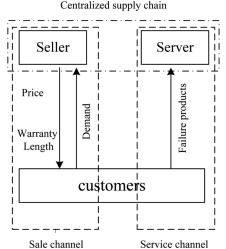Figure 1. The proposed supply chain model.

The sales price and warranty length are determined in the sales channel to attract customer demand. The service channel serves the customers for repairing the failed products. We assume that customer demand depends on both price and warranty. We also assume that the firm sells a single product and there is a single server to repair the failed products.

The required notations to formulate the problem are defined as follows.

Parameters:

• ${\textstyle \lambda }$ : The expected failure rate of products per unit of time;
• ${\textstyle C_{r}}$ : The expected repair cost for each failure;
• ${\textstyle C_{\mu }}$ : The unit cost of service capacity;
• ${\textstyle D}$ : Customer demand in the warranty period;
• ${\textstyle {\bar {\lambda }}}$ : Expected number of failures to be repaired in the warranty period.

Decision variables:

• P : The sales price of each product;
• W : The warranty length;
• ${\textstyle \mu }$ : The service capacity of server for after sales services.

The profit function contains revenue from selling the products and cost for carrying out the after sales service. Since the seller sells D   products in the warranty period, its revenue is ${\textstyle P{_{\ast }}D}$ . The expected number of failures is ${\textstyle {\bar {\lambda }}=\lambda {_{\ast }}W{_{\ast }}D}$ , and the repairing cost in the warranty period is ${\textstyle C_{r}{_{\ast }}{\bar {\lambda }}}$ . The cost of constructing a service center with capacity ${\textstyle \mu }$ is ${\textstyle C_{\mu }{_{\ast }}\mu }$ . Therefore, the profit function is as Eq. (1) :

 $Z_{1}=P\cdot D-C_{r}\cdot {\bar {\lambda }}-C_{\mu }\cdot \mu {\mbox{.}}$
( 1)

The second objective is related to minimizing the expected waiting time to receive the after sales services. The queuing system based on assumption is ${\textstyle M/M/1}$ . Therefore, the expected waiting time is as Eq. (2)    :

 $Z_{2}={\frac {1}{\mu -{\bar {\lambda }}}}{\mbox{.}}$
( 2)

The mathematical programming model can be written as follows:

 ${\begin{array}{c}{\mbox{ Max:}}\quad Z_{1}=P\cdot D-C_{r}\cdot {\bar {\lambda }}-C_{\mu }\cdot \mu \\{\mbox{Min:}}\quad Z_{2}={\frac {1}{\mu -{\bar {\lambda }}}}\\Subject~to{\mbox{:}}\\P>{\frac {C_{r}\cdot {\bar {\lambda }}+C_{\mu }\cdot \mu }{D}}\\\mu >{\bar {\lambda }}{\mbox{,}}\\W>0\end{array}}$
( 3)

where the constraint ${\textstyle P>{\frac {C_{r}\cdot {\bar {\lambda }}+C_{\mu }\cdot \mu }{D}}}$ ensures the non-negativity of the profit function and ${\textstyle \mu >{\bar {\lambda }}}$ ensures the stability condition of the queuing system.

## 3. Fuzzy expert system to estimate the demand

Demand estimation is one of the key factors in revenue management. There is no mathematical function to estimate the exact demand value. In the literature, there are many approaches that approximate the demand function based on deterministic, stochastic or fuzzy assumptions. In this article, we propose a fuzzy system that uses direct expert knowledge to estimate the demand. This fuzzy system is directly incorporated into a genetic algorithm that solves the problem.

In many real cases, decision making and problem analyzing is based on human knowledge and expert judgment. To analyze imprecise data which are not easily incorporated into existing analytical methods, fuzzy logic is among the most well-known. The fuzzy logic approach, which was introduced by Zadeh   ,   and  , is equal to “Computing with words” and is used to formulate human knowledge with linguistic terms. In this paper, a fuzzy system is designed to estimate the demand value. Since the demand value affects both profit and queue, the problem is to optimize the sales price and warranty length to have an optimal demand rate. After designing the fuzzy system, we utilize an optimization loop of an integrated genetic algorithm and a fuzzy system to obtain the optimal decision variables. In the following, the components of the fuzzy system are illustrated.

### 3.1. Fuzzy rule base

The linguistic terms utilized in this paper include: very low (VL), fairly low (FL), low (L), medium (M), high (H), fairly high (FH), and very high (VH). The low, fairly low, medium, high, and fairly high linguistic terms are applied as triangular fuzzy numbers. The linguistic variables of very low and very high are formulated as trapezoidal fuzzy numbers. The membership functions of triangular and trapezoidal fuzzy numbers are as Eqs.  and  , respectively.

 $\mu _{X}(x)=\lbrace {\begin{array}{cc}(x-a)/(b-a){\mbox{;}}&a\leq x
( 4)

Our proposed fuzzy system contains two input variables of price and warranty length and one output variable of demand value as an MISO system. In order to design the fuzzy system, we partition the input variables into five linguistic terms, and the output variable into seven linguistic terms. The input and output variables are shown in Figure 2 .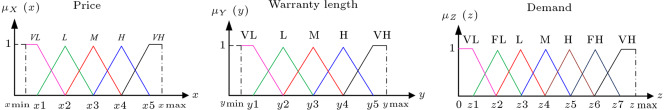Figure 2. Fuzzy partition of inputs and output universe set.

We use the if-then type rule in our proposed fuzzy rule base, which is in the following general form:

If price is 〈linguistic term〉 and warranty length is 〈linguistic term〉, then, demand is 〈linguistic term〉”.

Regarding the five linguistic terms for both input variables, we have 25 rules in our proposed rule base. The fuzzy rule base derived from expert knowledge is shown in Table 1 .

Table 1. Fuzzy rule base.
Rule number If part Then part
Price Warranty length Demand
1 VL VL M
2 VL L H
3 VL M FH
4 VL H VH
5 VL VH VH
6 L VL M
7 L L H
8 L M H
9 L H FH
10 L VH FH
11 M VL L
12 M L M
13 M M M
14 M H H
15 M VH H
16 H VL FL
17 H L L
18 H M L
19 H H M
20 H VH M
21 VH VL VL
22 VH L FL
23 VH M FL
24 VH H L
25 VH VH M

### 3.2. Fuzzy inference engine

We use the Mamdani implication mechanism as an inference system in our fuzzy system. The fuzzy singleton fuzzifier is used in the fuzzification section of the fuzzy system and the centroid method for deffuzification   . Assuming that ${\textstyle x_{0}}$ and ${\textstyle y_{0}}$ are the fuzzy singleton of the price and warranty length, the fuzzy inference is applied by the following steps:

Step 1: Map the input fuzzy singletons into their universe sets.

Step 2: Combine input fuzzy singletons and obtain the active rules.

Step   3: Calculate ${\textstyle \mu _{X}(x_{0})}$ and ${\textstyle \mu _{Y}(y_{0})}$ using Eqs.  and  , and denote them by ${\textstyle h_{1}}$ and ${\textstyle h_{2}}$ , respectively.

Step   4: Determine the matching degree (${\textstyle \alpha _{j};j=1,\ldots ,J}$ ) for each active rule as

 $\alpha _{j}=min(h_{1},h_{2}){\mbox{.}}$
( 6)

Step   5: Execute the Max–Min operator to determine the outputs (${\textstyle u_{j}^{'}}$ ).

Step 6: Repeat steps 3–5 for all active rules. Figure 3 indicates the inference steps for a sample active rule.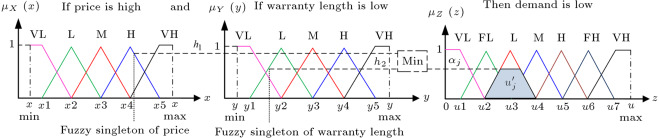Figure 3. Mamdani inference system.

Step   7: Convert fuzzy outputs (${\textstyle u_{j}^{'}}$ ) into the crisp values by centroid defuzzification using Eq. (7) . Let ${\textstyle {\bar {u}}^{j}}$ be the center of ${\textstyle u_{j}^{'}}$ :

 $u_{0}={\frac {\sum _{j=1}^{J}{\bar {u}}^{j}\times \alpha _{j}}{\sum _{j=1}^{J}\alpha _{j}}}{\mbox{,}}$
( 7)

where ${\textstyle u_{0}}$ is the demand value that is calculated by the fuzzy system.

## 4. ${\textstyle L{\mbox{–}}p}$ metric technique4. L – p {\textstyle L{\mbox{–}}p} metric technique

Since we maximize seller profit as well as minimize waiting time in the after sales services center, the problem is a multi objective optimization problem. The general model of a multi objective optimization problem is as follows   :

 $max:F(x)=\lbrace f_{1}(x),f_{2}(x),\ldots ,f_{k}(x)\rbrace {\mbox{,}}$
( 8)

where k   is the number of objectives, (${\textstyle k>1}$ ), and ${\textstyle X\subseteq R^{k}}$ is the feasible solution space. Converting the multi objective problem into a single objective problem is one of the usual methods in MODM techniques   . In this research, the ${\textstyle L{\mbox{–}}p}$ metric technique is used to solve the bi-objective problem. The main approach of this technique is minimizing the difference between each objective function with its individual optimum value, and also choosing the appropriate value for each objective which is the nearest point to the individual optimum solution. The model of the ${\textstyle L{\mbox{–}}p}$ metric technique was developed as Eq. (9)    :

 ${\mbox{Min: }}L{\mbox{–}}p=\lbrace \sum _{j=1}^{k}\gamma _{j}[{\frac {f_{j}(x^{_{\ast }})-f_{j}(x)}{f_{j}(x^{_{\ast }})}}]^{t}\rbrace ^{1/t}{\mbox{,}}$
( 9)

where ${\textstyle \gamma _{j}}$ is the weight of objective function j   and ${\textstyle f_{j}(x^{_{\ast }})}$ is the individual optimum value of function j   . The value of ${\textstyle t(1\leq t\leq \infty )}$ presents the stress degree of difference between the individual optimum values and the efficient solution of the problem.

## 5. Hybrid solution of genetic algorithm and fuzzy expert system

In this section, at first, we describe the proposed Genetic Algorithm (GA), and then integrate it with the fuzzy system to construct the hybrid solution method.

### 5.1. The proposed genetic algorithm

The Genetic Algorithm (GA) is one of the best evolutionary algorithms introduced by Holland    and  . The efficiency of GAs is shown in the various areas of operational and industrial applications to optimize the problem   . The GA starts with a randomly created initial set of solutions (initial population). Each solution in the population is named a chromosome and each chromosome includes several genes, where each gene indicates a decision variable of the problem. Then, applying the crossover and mutation operators, a new generation is produced. During each generation, the chromosomes are evaluated with a fitness function. The procedure is repeated till the stop criterion is satisfied.

In this paper, the chromosome structure is defined as Figure 4 .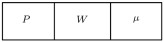Figure 4. Structure of chromosome.

In the proposed chromosome, genes P , W   and ${\textstyle \mu }$ are price, warranty length, and retail rate of service, respectively. P and W genes are related to the fuzzy system input variables.

The steps of the proposed GA are illustrated as follow:

Step 1. Initial population

The initial population is generated randomly considering an interval for each decision variable. Considering Eqs. (3)  and  , the fitness value of each chromosome is calculated.

Step 2. Parent selection

The rank based proportional selection method and the roulette wheel method are used for choosing the parents, in order to implement the cross over or mutation operators.

Step 3. Crossover operator

We use the uniform crossover operator on two selected parents. The genes for applying the crossover operator are selected via a random binary variable. The value of each gene that is one is exchanged to zero. Figure 5 illustrates the crossover operator.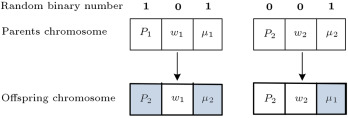Figure 5. A sample of crossover.

Step 4. Mutation operator

To apply the mutation operation, several genes of a selected chromosome are chosen randomly and their values are changed.

Step 5. The replacement

We use the tournament replacement method to select a group of chromosomes at each step and replace the two worst chromosomes with two new generated ones.

### 5.2. Hybrid solution method of genetic algorithm and fuzzy expert system

Finally, we integrate the proposed GA with the fuzzy system to develop a hybrid solution method. The role of the fuzzy system is determining the demand value based on variables determined by GA. The procedure of the proposed hybrid solution method is represented in Figure 6 .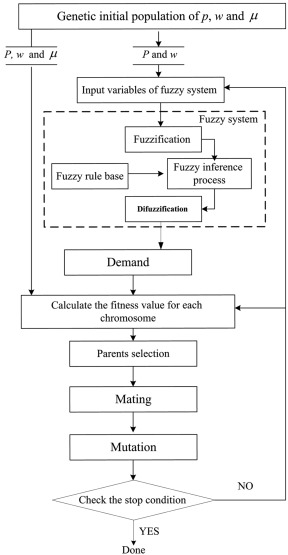Figure 6. The hybrid solution of GA and fuzzy system.

## 6. Computational results

In this section, a numerical example is illustrated to show how the hybrid solution method determines the demand value, as well as suitable values of decision variables. The partitioning of price and warranty length as input variables of the fuzzy system, and demand as the output variable, are shown in Figure 7 . The fuzzy singletons of input variables, including price and warranty length, are 900 and 5, respectively. Table 2 shows the active rules and demand value determined by the rule base.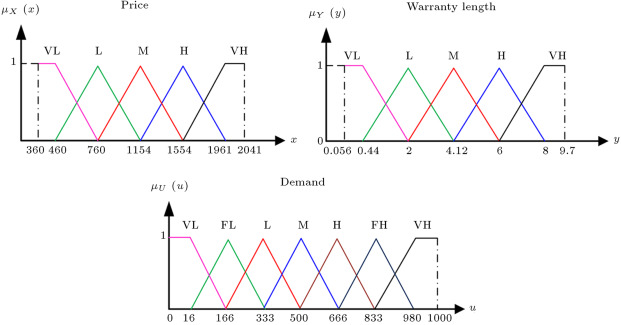Figure 7. The fuzzy partition of inputs and output variables in the numerical example.

Table 2. Active rules and inference results of numerical example.
Rule number If part Then part ${\textstyle \alpha _{j}}$ ${\textstyle {\bar {u}}^{j}}$ ${\textstyle \alpha _{j}\times {\bar {u}}^{j}}$ ${\textstyle \sum _{j=1}^{4}\alpha _{j}}$ ${\textstyle \sum _{j=1}^{4}{\bar {u}}^{j}\times \alpha _{j}}$ ${\textstyle u_{0}}$
P W D
8 L M H 0.53 666 352.98 1.69 1144.26 677.07
9 L H FH 0.46 833 383.18
13 M M M 0.35 500 175
14 M H H 0.35 666 233.1

Figure 8 shows how the demand value changes with respect to price and warranty length. In fact, this figure shows that the performance of the rule base and inference mechanism is reasonable and acceptable regarding application.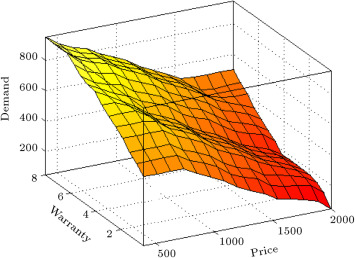Figure 8. The behavior of demand by changing both price and warranty.

The numerical example is continued by applying the hybrid algorithm to determine the best value for decision variables. Assume that ${\textstyle C_{r}=40,C_{\mu }=150,\lambda =1}$ , ${\textstyle t=2}$ and ${\textstyle \gamma _{1}=3}$ and ${\textstyle \gamma _{2}=1}$ . In order to calculate the efficient solution, first, the individual optimum solution of profit and waiting time is obtained. Next, we achieve an efficient solution using the ${\textstyle L{\mbox{–}}p}$ metric technique. After implementing the hybrid solution of GA and fuzzy logic, with ${\textstyle Z_{1}}$ and ${\textstyle Z_{2}}$ as the cost function of GA, individual optimum values are ${\textstyle Z_{1}^{_{\ast }}={\mbox{38 0071}}}$ and ${\textstyle Z_{2}^{{_{\ast }}^{'}}=2020(Z_{2}^{_{\ast }}=0.000495)}$ . Therefore, the ${\textstyle L{\mbox{–}}p}$ metric model to find the efficient solution is as follows:

 ${\mbox{Min: }}L{\mbox{–}}p=\lbrace 3\times [{\frac {Z_{1}^{_{\ast }}-Z_{1}}{Z_{1}^{_{\ast }}}}]^{2}+1\times [{\frac {Z_{2}^{{_{\ast }}^{'}}-Z_{2}^{'}}{Z_{2}^{{_{\ast }}^{'}}}}]^{2}\rbrace ^{1/2}{\mbox{.}}$

We use the objective function, ${\textstyle MaxZ_{2}^{'}=1/Z_{2}}$ , instead of ${\textstyle MinZ_{2}}$ to have the two objectives in the form of maximization. The hybrid solution method of GA and fuzzy logic is performed in Matlab software to minimize the ${\textstyle L{\mbox{–}}p}$ metric model and find the best values of decision variables. The results for 10 runs of GA are shown in Table 3 .

Table 3. Genetic algorithm outputs.
Run number Decision variable Objective 1: ${\textstyle Z_{1}}$ Objective 2: ${\textstyle Z_{2}}$ ${\textstyle L{\mbox{–}}p}$  metric
P W ${\textstyle \mu }$ Profit Waiting time  ${\textstyle x10^{3}}$
1 1105 0.54 753 322365 1.869 0.3394
2 1052 0.72 860 322625 1.841 0.3358
3 1078 0.64 806 325940 1.862 0.3304
4 1399 2.07 1400 318243 1.758 0.3375
5 1024 0.05 539 320970 1.923 0.3483
6 1403 2.10 1337 326990 2.003 0.3420
7 1434 1.98 1358 319947 1.682 0.3241
8 1405 2.03 1299 333687 2.043 0.3317
9 1095 0.58 794 298437 1.799 0.3351
10 1078 0.64 796 327355 1.900 0.3312

In this sample, the best solution is obtained in run 7. To show the intelligence of the proposed GA, we plot the convergence diagram in Figure 9 .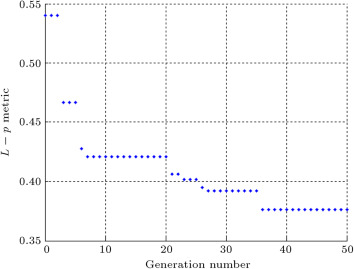Figure 9. Convergence diagram of genetic algorithm.

We generated 20 sample problems to analyze the performance of the problem and gain the numerical results. To analyze the robustness of the efficient solution, we differentiated the objectives weight between the sample problems and tabulated the results in Table 4 . The results show the robustness of the best solution and also a set of efficient solutions.

Table 4. Obtained efficient solutions by various weights of objective functions.
Problem no Weight of objective function 2 is fixed and equal one (${\textstyle \gamma _{2}=1}$ )
Weight of objective function 1 (${\textstyle \gamma _{1}}$ ) Objective value
Profit Waiting time
1 1 250140 1.017
2 2 294724 1.449
3 3 319947 1.689
4 4 329802 1.923
5 5 339463 2.465
6 6 347564 2.944
7 7 349996 3.052
8 8 353664 3.413
9 9 357504 3.617
10 10 358634 3.700
Problem no Weight of objective function 1 is fixed and equal one (γ1=1)
Weight of objective function 2 (γ2) Objective value
Profit Waiting time
11 1 250140 1.017
12 2 195757 0.783
13 3 184326 0.684
14 4 150490 0.597
15 5 142159 0.578
16 6 141039 0.567
17 7 135627 0.557
18 8 124983 0.548
19 9 122410 0.537
20 10 121439 0.527

The behaviors of objective functions, with respect to changes in weight of objective functions, are also illustrated in Figure 10  and Figure 11 , based on the results of Table 4 .Figure 10. The behavior of first objective function by changing the weight of both objective functions.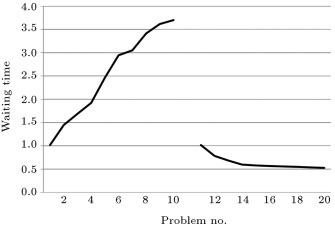Figure 11. The behavior of second objective function by changing the weight of both objective functions.

In order to gain more insight into how the model responds to the changes in the failure rate, we study the sensitivity of each objective function against the failure rate. As expected, the results show that both objective functions cannot be improved by increasing the failure rate (Figure 12  and Figure 13 ).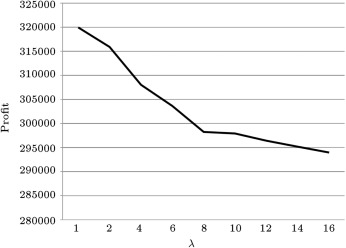Figure 12. The behavior of profit function by changing the failure rate.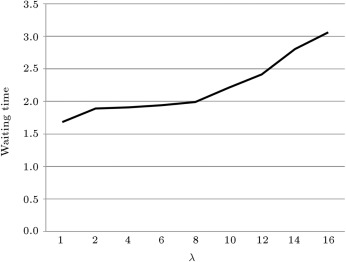Figure 13. The behavior of waiting time function by changing the failure rate.

## 7. Conclusion

In this paper, we investigated the warranty length, price, and capacity rate of a service center by a mathematical model in a queuing framework. We considered two objective functions of maximizing profit and minimizing the waiting time of customers in after sales service systems. We also applied the ${\textstyle L{\mbox{–}}p}$ metric technique to analyze the bi-objective optimization problem and proposed a hybrid solution method of a GA and a fuzzy system to solve the model. The fuzzy system is carried out to estimate customer demand, based on price and warranty length obtained by a GA. The main contribution of this paper is developing a fuzzy system to estimate demand value and its integration with a genetic algorithm to construct a hybrid solution method. We illustrate the performance of our proposed genetic algorithm and demand estimation technique based on a fuzzy system in a numerical study. Our results show that both proposed techniques perform quite well. A sensitivity analysis on failure rate has also been done, and the results show that both objective functions are worst in cases of increasing failure rate. Potential future research can be towards developing a Pareto-based evolutionary algorithm, such as a non-dominated sorting genetic algorithm, or multi-objective particle swarm optimization to find the Pareto front solutions.

### Document informationPublished on 06/10/16

Licence: Other

### Document Score0

Views 9
Recommendations 0